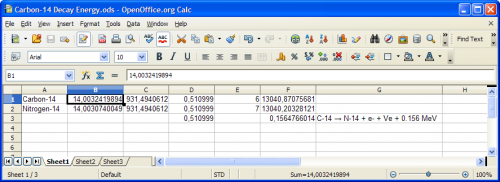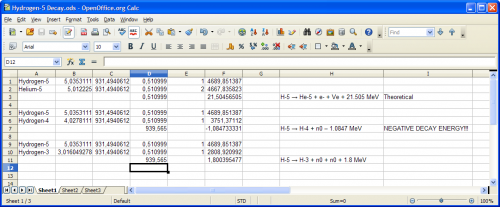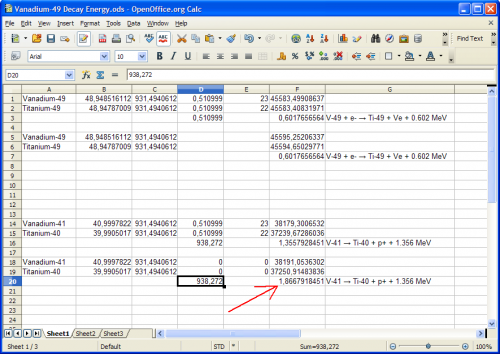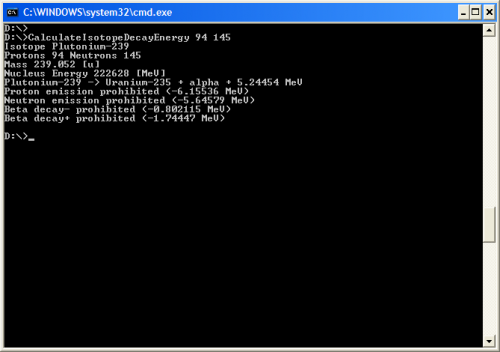# Radioactive decay and information (split from what is real in physics)

## Recommended Posts

if everything is information, the randomness of radioactive decay could be explained by the mechanism of description of individual atoms. If every atom is a specific algorithm set, consisting of strings of numbers at it's most fundamental level, perhaps the algorithm contains largely similar number sets, with small variations, like junk DNA in living cells. One or two variations in a cell's DNA might have no significant bearing on the identity or functioning of a particular cell. Similarly, small variations in protons and neutrons mathematical makeup might cause the randomness of certain radioactive decays...yet have them remain as functioning particles in normal relationships with other fundamental particles. Their sub-components of quarks are likely simpler arrangements of numbers and have few variations within them, but added together in the 3 quark components of protons or neutrons, the cumulative effects add complexity to the situation for each individual atom. If this is true, then a direct readout of the complete algorithmic structure of individual atoms could allow a prediction of the life span of a particular atom, and make the radioactive decay more predictable...ps to peter, if we don't "go there" into mysticism, we won't de-mystify it...

Edited by hoola

##### Share on other sites

…and the connection to what's real in physics is…

##### Share on other sites

The basis of this connection with physics is we use math measurements in physics and in a sense math is measuring itself, or rather completing an analysis of it's own internal makeup. I will start a new thread...

##### Share on other sites

if everything is information, the randomness of radioactive decay could be explained by the mechanism of description of individual atoms. If every atom is a specific algorithm set, consisting of strings of numbers at it's most fundamental level, perhaps the algorithm contains largely similar number sets, with small variations, like junk DNA in living cells. One or two variations in a cell's DNA might have no significant bearing on the identity or functioning of a particular cell. Similarly, small variations in protons and neutrons mathematical makeup might cause the randomness of certain radioactive decays...yet have them remain as functioning particles in normal relationships with other fundamental particles. Their sub-components of quarks are likely simpler arrangements of numbers and have few variations within them, but added together in the 3 quark components of protons or neutrons, the cumulative effects add complexity to the situation for each individual atom. If this is true, then a direct readout of the complete algorithmic structure of individual atoms could allow a prediction of the life span of a particular atom, and make the radioactive decay more predictable...ps to peter, if we don't "go there" into mysticism, we won't de-mystify it...

Do you know how to calculate Decay Energy of unstable radioactive isotope?

First, find parent isotope mass. They're in f.e. wikipedia articles such as http://en.wikipedia.org/wiki/Isotopes_of_hydrogen (last portion of link is name of element).

For instance Carbon-14 has 14.0032419894 u

Then, you need to multiply it by 1u = 931.494 MeV to receive total energy of nucleus + electrons.

Then you need to get rid of mass-energy of electrons. Multiplying 0.510999 MeV by quantity of protons/electrons. Subtract total energy of electrons from energy of isotope.

Repeat it for daughter isotope. In Carbon-14 case it's Nitrogen-14.

Subtract N-14 nucleus energy from C-14 nucleus energy.

Subtract electron energy (it's emitted during decay together with antineutrino)

Result will be Decay Energy of isotope (kinetic energy of particles + energy of neutrino in our case).

Decay Energy must be positive value.

If you will calculate Decay Energy from stable isotope such as Deuterium, Helium-3, Helium-4 for all possible decay modes you will see that D.E. would be negative value. Thus these particles are stable.

For Carbon-14 it's 0.156 MeV.

As you can see this value does match article:

http://en.wikipedia.org/wiki/Carbon-14Other examples:Fusion of proton-proton, decay of carbon-11 and decay of Mangan-54:I wrote computer application which has built-in database of isotopes and calculates possible decay modes and released energy.

##### Share on other sites

Hoola - For some reason I cannot quote your post. I thought it was very interesting and made sense, as much as any information theory does. I have no idea whether your suggestion would count as a scientific hypothesis. David Chalmers seems to think so, but it sounds as if Swansont has an objection. Among its proponents would be the Buddha and Lao-tzu, so if it is a scientific hypothesis this would be strange.

ps. That was a most surprising ps. I agree of course.

.

##### Share on other sites

Then you need to get rid of mass-energy of electrons. Multiplying 0.510999 MeV by quantity of protons/electrons. Subtract total energy of electrons from energy of isotope.

The electron mass is only an issue for beta plus decays, where the total number of electron-mass particles changes. Otherwise they cancel in the atomic mass measurements. The change in electron/atomic binding energy of the system is a few eV, which can be ignored.

##### Share on other sites

The electron mass is only an issue for beta plus decays, where the total number of electron-mass particles changes.

Not only.

Proton emission also, double proton emission.

It's easy to make mistake that will remain unnoticed if we will be doing such shortcuts.(in above example one electron and one proton will be free)

##### Share on other sites

Not only.

Proton emission also, double proton emission.

It's easy to make mistake that will remain unnoticed if we will be doing such shortcuts.

It's not a shortcut. You account for all of the particles. Proton emission is not an exception. You use the mass of H-1.

In my experience it's more likely you'll make a mistake making the equation more unwieldy by adding and subtracting terms that cancel (especially with some folks' tendency to calculate numbers instead of simplifying formulas)

##### Share on other sites

In application I have functions:

double get_isotope_mass( int protons, int neutrons ); // read isotope database and return mass in units

double get_isotope_energy( int protons, int neutrons ); // call above, multiply by 931.494 MeV etc. return energy in [eV]

double get_nucleus_energy( int protons, int neutrons ); // call above, and subtract electrons energy (protons * 0.5109989). return energy in [eV]

All other code is only using get_nucleus_energy().

I wrote application, to not have to worry about calcs.

Simply enter protons,neutrons quantity and app is displaying what are possible decay modes and Decay Energy.

For instance decay of Plutonium-239 is prohibited in all modes but alpha decay,

alpha decay energy 5.24454 MeV does match article

http://en.wikipedia.org/wiki/Plutonium-239Edited by Sensei

##### Share on other sites

I wrote application, to not have to worry about calcs.

That's great. But to anyone who doesn't have an application and is using a calculator, a more compact calculation is preferable.

##### Share on other sites

See how easy is to introduce error.

Check Zn-64 on wiki

http://en.wikipedia.org/wiki/Isotopes_of_zinc

They say it's "observatory stable" with possible double beta decay+

But calculate decay energy, and it'll be obvious it cannot happen.

Simply sum of nucleus of Ni-64 + 2e+ have higher mass-energy than nucleus Zn-64.

They had to use D.E.=(mparent-mchild)*931.494 MeV=73.6 keV

This error is repeated over and over again in many elements.

I have checked three elements, and all three wrong.

Ni-58 another example

http://en.wikipedia.org/wiki/Isotopes_of_nickel

##### Share on other sites
On 14 June 2014 at 9:27 PM, Sensei said:

See how easy is to introduce error.

Check Zn-64 on wiki

They say it's "observatory stable" with possible double beta decay+

But calculate decay energy, and it'll be obvious it cannot happen.

Simply sum of nucleus of Ni-64 + 2e+ have higher mass-energy than nucleus Zn-64.

They had to use D.E.=(mparent-mchild)*931.494 MeV=73.6 keV

This error is repeated over and over again in many elements.

I have checked three elements, and all three wrong.

Ni-58 another example

Why is Wiki wrong

& repeatedly making

the same mistake?

(Don't they know what they are doing?)

Can they be helped

to be put (back) on (the right) track again?

I don't think anybody wants wrong info,

not even them.

Where has the error started?

How can this mess be corrected, for good?

##### Share on other sites

Generally, when you see "Observatory Stable" on wikipedia on Isotopes websites, theory states that some isotope should decay, but they don't want to decay in human enough to bother observation times.

Edited by Sensei

##### Share on other sites
On 6/14/2014 at 3:27 PM, Sensei said:

But calculate decay energy, and it'll be obvious it cannot happen.

Simply sum of nucleus of Ni-64 + 2e+ have higher mass-energy than nucleus Zn-64.

Where did you find the masses of these nuclei?

##### Share on other sites

This argument is silly, there is an equation that explains this, exactly, move this to speculations like you do every other post like this.##### Share on other sites
8 hours ago, Vmedvil said:

This argument is silly, there is an equation that explains this, exactly, move this to speculations like you do every other post like this.We were discussing Q value, not activity, and there is no speculation here.

##### Share on other sites
6 hours ago, swansont said:

We were discussing Q value, not activity, and there is no speculation here.

Oh, I didn't read the rest of the post just the first post by the OP, okay then.

## Create an account

Register a new account